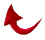And the Miracle Continues... All Praise Be to God.

"By the dawn. And the ten nights. By the even and the odd. By the night as it passes.  A profound oath, for one who possesses intelligence" (89:1-5).

The Quran's 19-basedmathematical miracle continues to manifest itself in different forms as time goes on. God keeps sending signs to the faithful that the Ouran is His unaltered word and no falsehood can enter it, and to strengthen their faith as stated in Sura 74:31.

The miracle that I will be trying to explain to you is based on a single verse in the Ouran where the focus is placed on only two words! The verse is 89:3 from the chapter called the Dawn. In this verse: God swears by THE EVEN AND THE ODD. At first, this may sound meaning­less to a casual reader of the Quran. How­ever, to a sincere student of the Quran it is full of wonders and appreciation for God's power. Let me explain how.

Note that 893 is a multiple of 19. As a matter of fact, it is 47 x 19 So far in all investigations of Quran's mathematical miracle we have not looked at the properties of the integer which is the "quotient”.. In this case 47 happens to also be a "Prime” number (a number which is only divisible by itself and one). The number 47 can be partitioned into 19 + 28. The number 28 is another interesting number mathematically because it is the second "perfectnumber in number theory. The first per­fect number is 6. Remember the number of chapters in the Ouran equals 114, which is 6 x 19. What are perfect numbers? A perfect number is a number that the sum and multiples of its factors are equal to itself. For example, 6 = 1 +2 +3 and also 6 = 1 x 2 x 3. As I mentioned above, the second perfect number is 28, and the third 496, and so on. Remember God created the heavens and the earth in "6 DAYS". He sent the Ouran in a PER­FECT ARABIC TONGUE. The second perfect number, which is 28, happens to be the number of Arabic alphabet letters.

Let me now go back to the EVEN and the ODD. Let us look at the number of verses in each chapter and see if it is odd or even. If we do this we find that there are 60 chapters in the Quran which possess an even number of verses and 54 which pos­sess an odd number of verses. Of course if you add these two numbers you obtain 114, as you should. However, the intricacy of the Quran's mathematical code be­comes more overwhelming if we examine these numbers as the indices of prime numbers. What do I mean by that? For example the 54th prime number is 251 and the 60th prime number is 281.  Now let us add them up.

251 + 281 = 532, and 532 = 28 x 19

This is only part of the story! Remember there are 112 verses in the Ouran which arc not numbered. These arc the Basmalah's which arc in the beginning of every chapter. The two exceptions arc Chapter 1 where the Basmalah is numbered and Chapter 9 where it is absent. What happens if we include these un-numbered verses in the total number of verses in each chapter? For example, Chapter 2 has 286 verses without the un-numbered Basmalah. If we include the Basmalah, the total number of verses should be 287. Chapter 3 has 200 verses without the Basmalah. The total number of verses would be 201 when we include the un-numbered Basmalah, and so on through Chapter 114. By counting the verses this way we obtain 52 chapters with an even number of verses and 62 chapters with an odd number of verses. The 52nd prime num­ber is 239 and the 62nd prime number is 293. Let's add them up.

239 + 293 = 532 and 532 = 28 x 19

Glory' be to God. How is that for a miracle? Do not relax. There is still more: We have two pairs of prime numbers 25l,281 and 239,293. Lets add up all these digits in these two pairs and see what we gel!

2 + 5 + 1 + 2 + 8 + 1 = 19

and

2 + 3 + 9 + 2 + 9 + 3 = 28

Again, absolutely awesome! The Al­mighty has designed the Quran in such a way which makes it obvious to the sincere that it is His word. God willing, with such a continuous miracle from God, as stated in the Quran, our faith will increase.

Dr. All R. FazelyReturn to Table of Contents